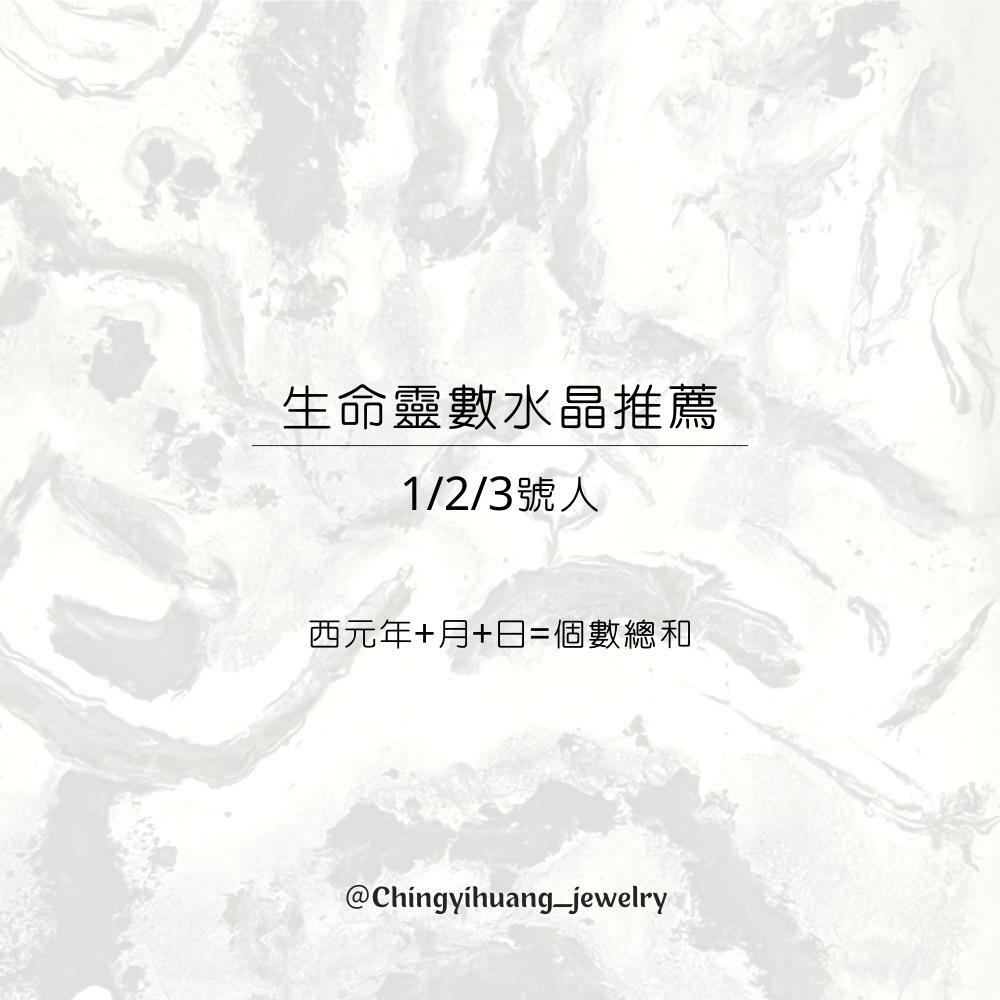A. 1988/01/01= 1+9+8+8+0+1+0+1=28
2+8=10
1+0=1號人

B. 1993/05/12= 1+9+9+3+0+5+1+2=30
3+0=3
3+0=3號人

C. 1995/03/15= 1+9+9+5+0+3+1+5=33
3+3=6號人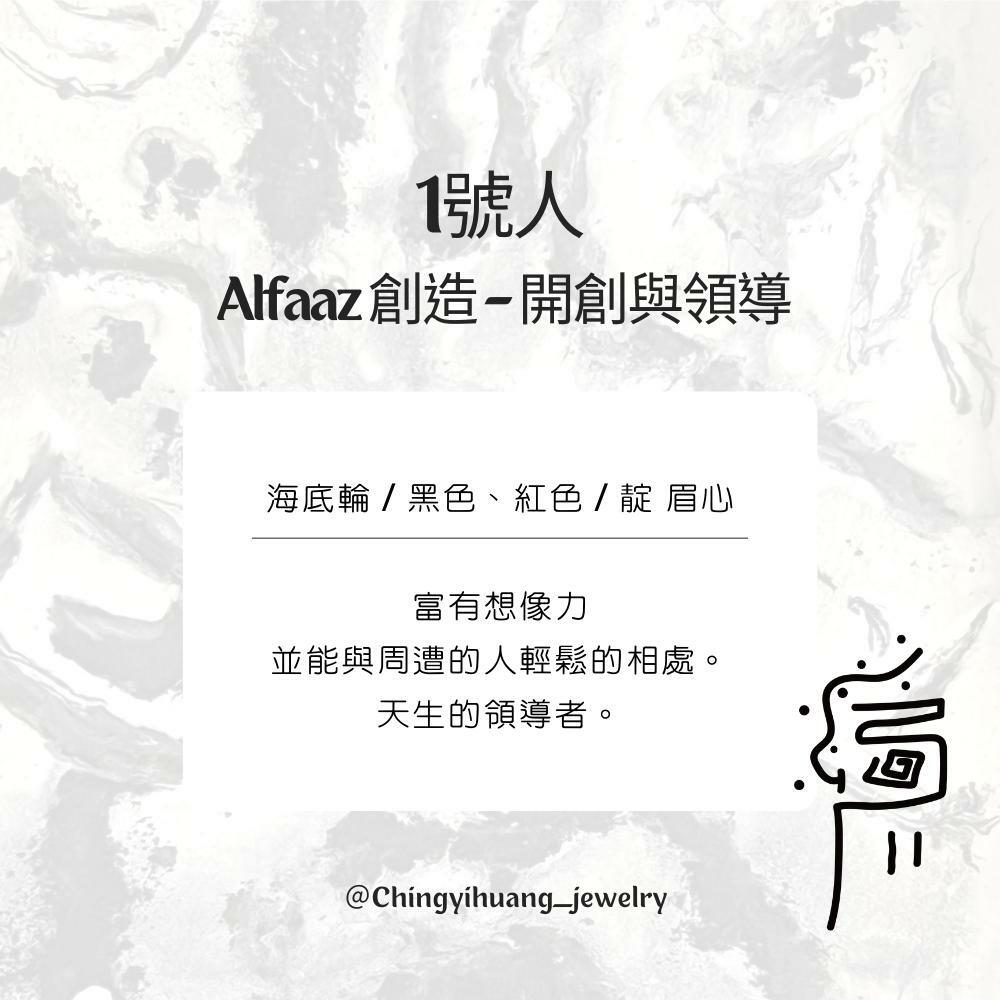1號人

Alfaaz 創造 – 開創與領導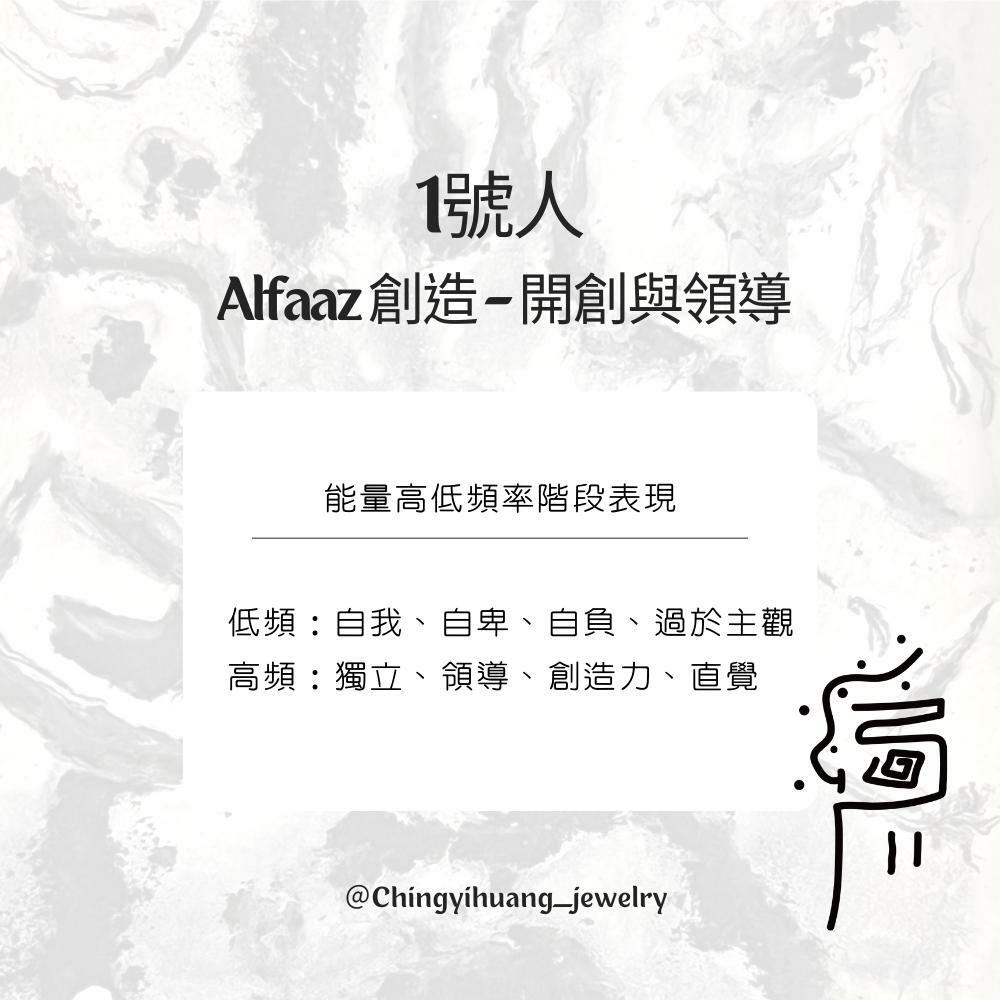🔳1號人 Alfaaz創造- 創造與領導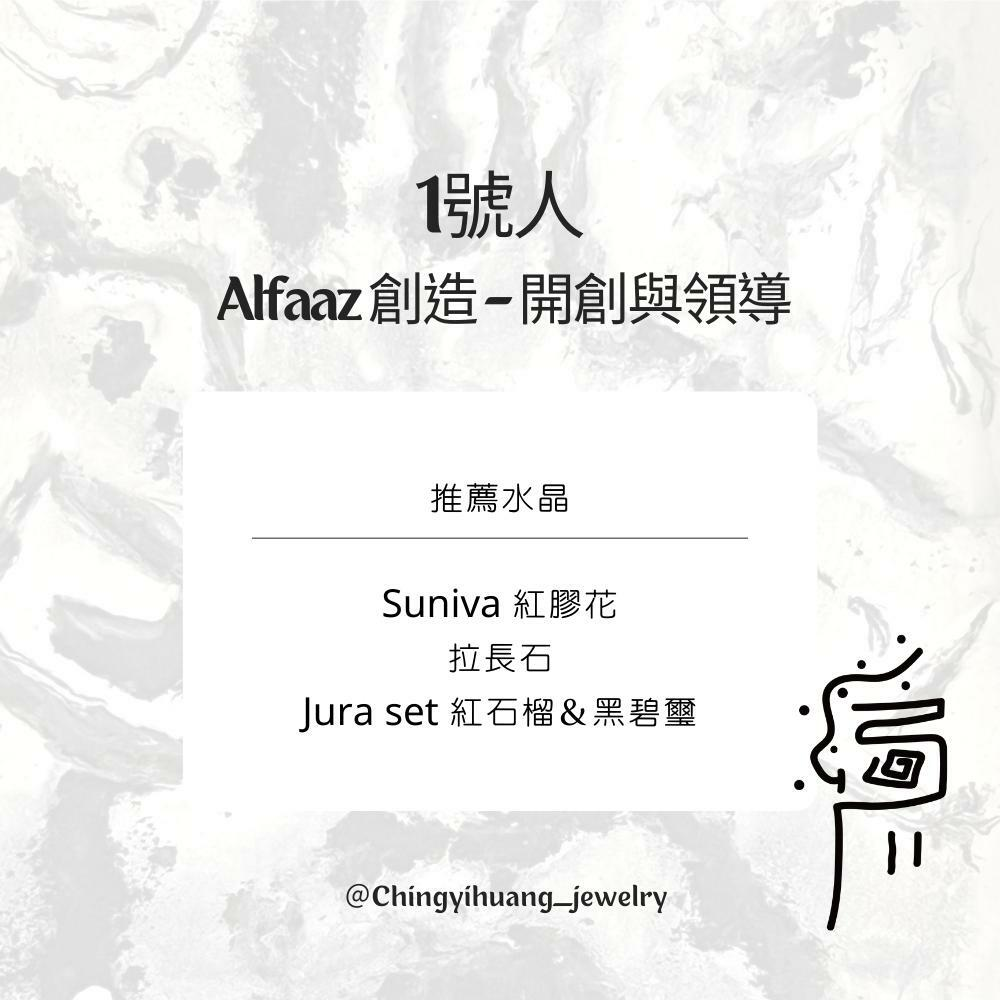CYH- Suniva 巴西 紅膠花 純銀 手環 (安神/招貴人/招桃花/正財/富足)

CYH- Jura set紅石榴＆黑碧璽 純銀 手環 (再生能力/女性賀爾蒙/消除負能量/海底輪)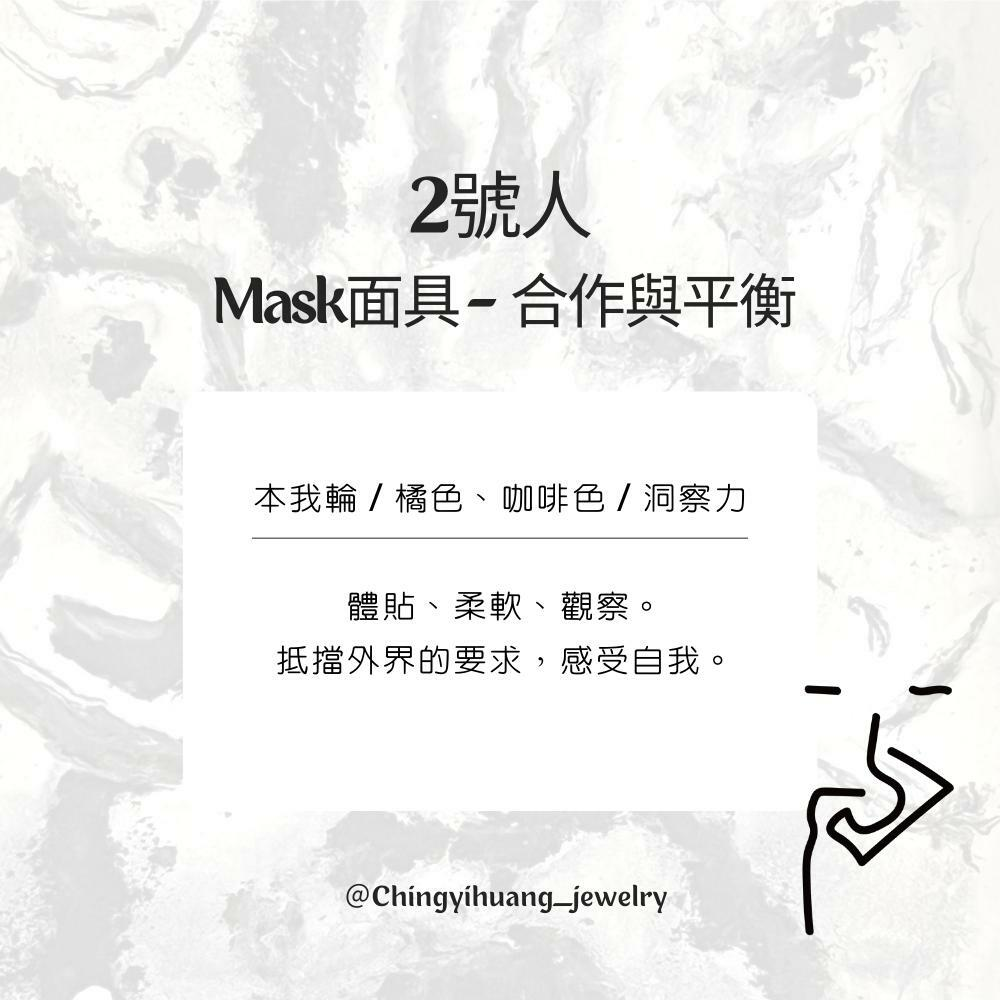2號人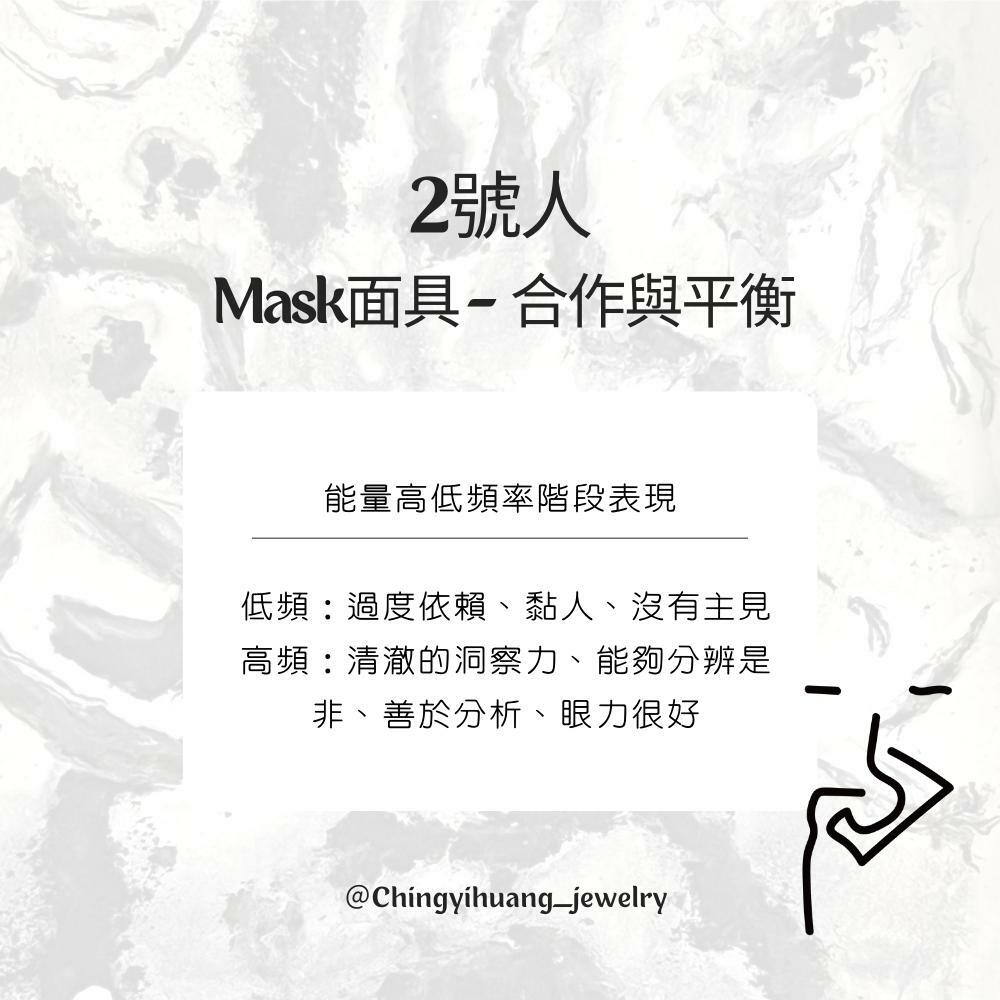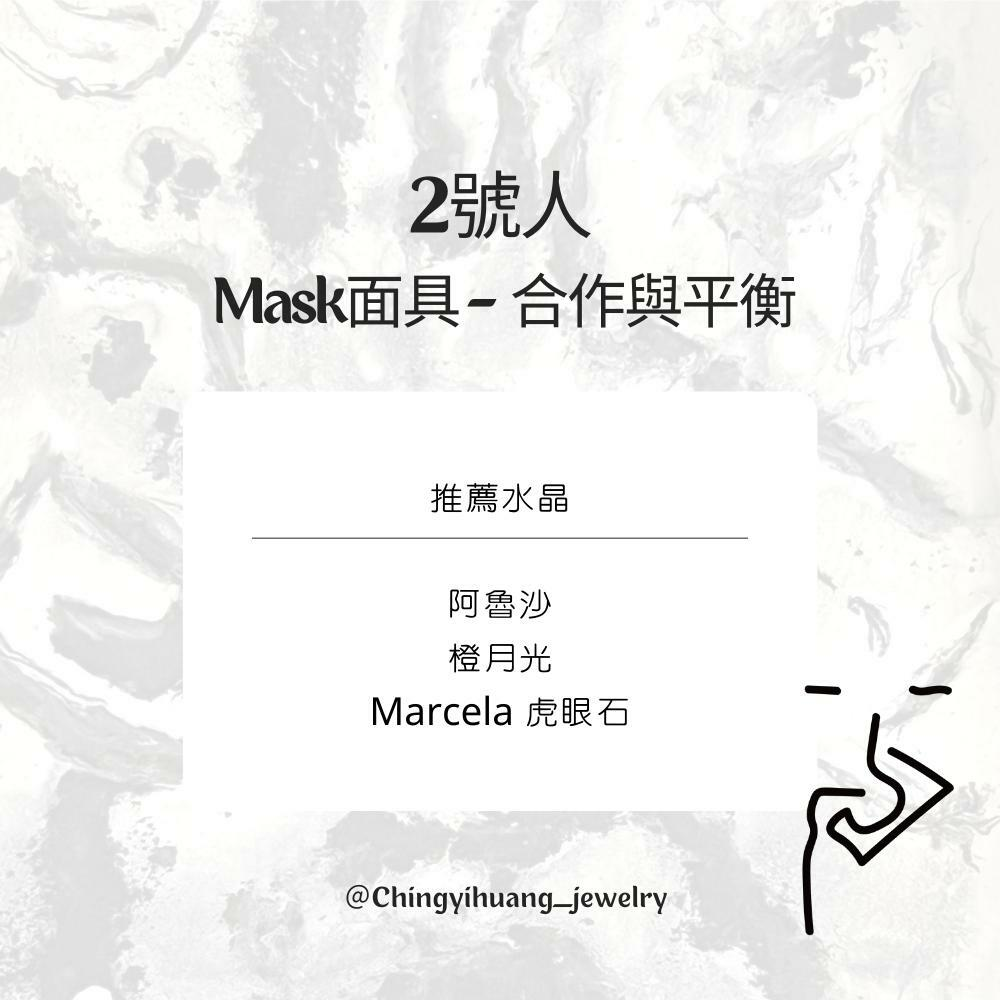CYH-天然 坦桑尼亞 阿魯沙 金太陽石/綠柱石 純銀 手環（正能量/帶來歡樂/對應太陽輪/心輪）

CYH-天然 斯里蘭卡 橙月光 水晶手環（ 健康/富貴/長壽/穩定情緒）

CYH – Marcela 虎眼石 純銀 手鍊（招財旺財/增加勇氣信心/阻擋外在負能量）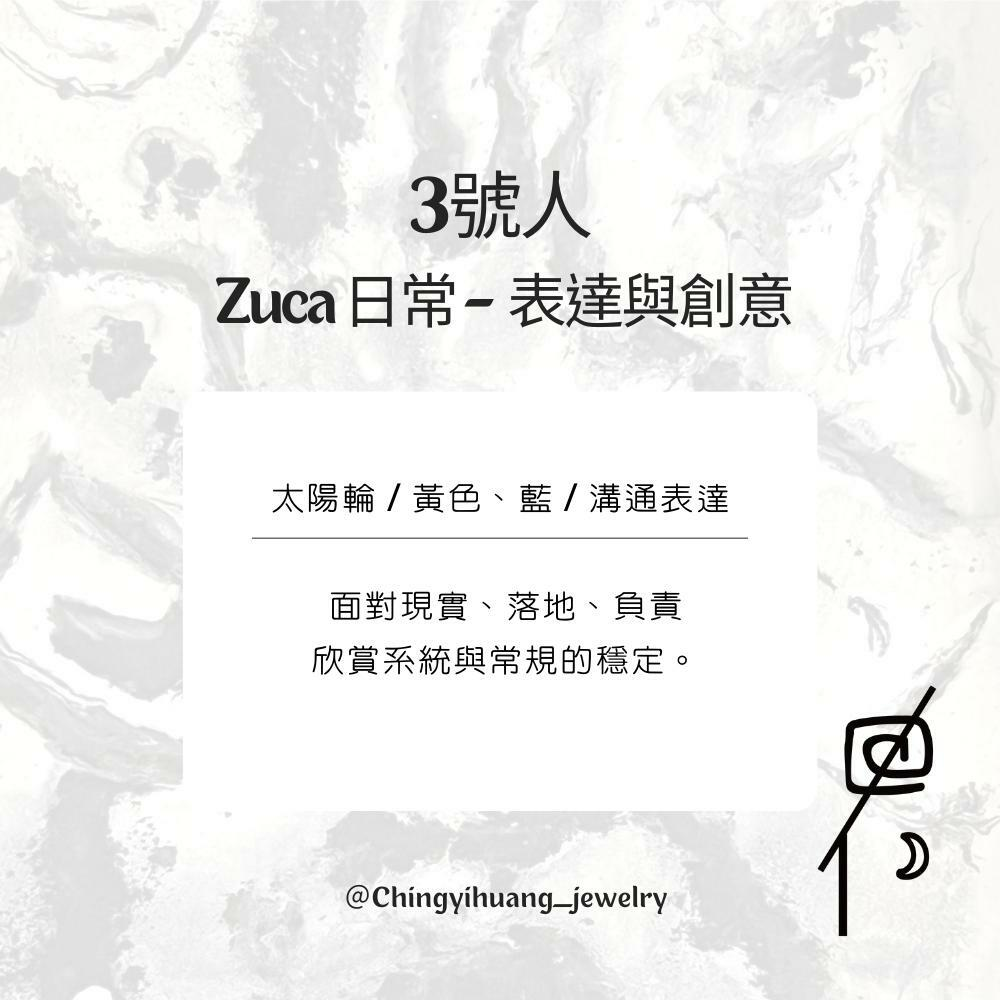3號人

Zuca 日常 – 表達與創意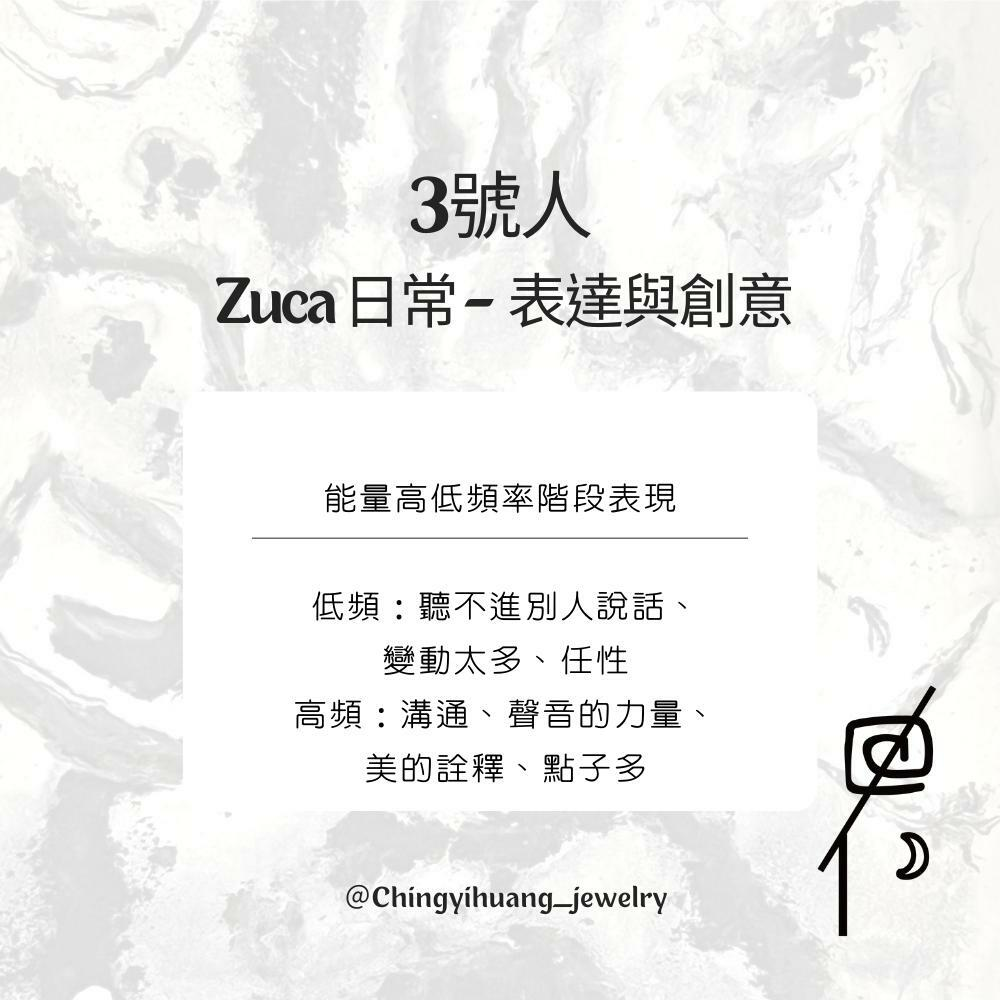🔳3號人 Zuca 日常- 表達與創意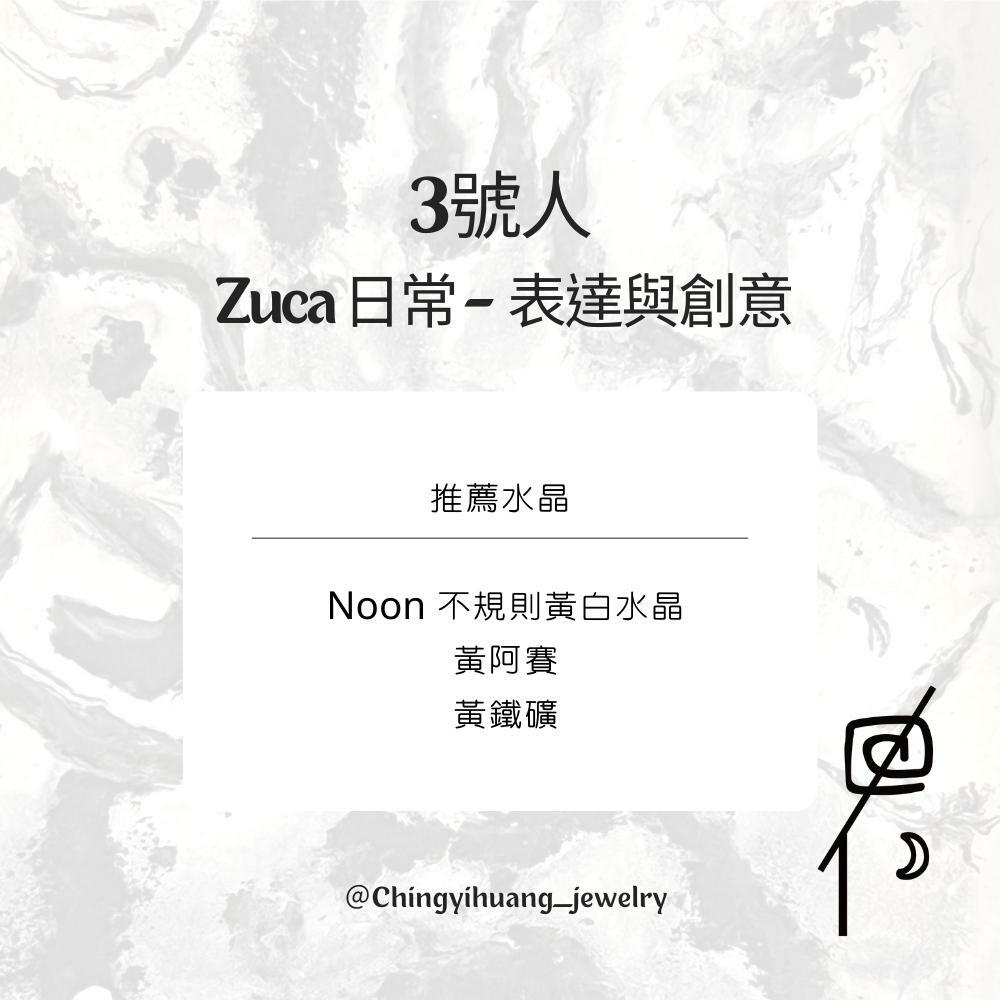CYH- Noon-不規則黃白水晶 純銀 手鍊 (增加財運/緩解緊張/增加自信/穩定事業)

CYH- 巴西 黃阿賽 手環 (招正財/精力充沛/緩解緊張/增加自信)

CYH-天然 巴西 黃鐵礦 純銀 項鍊（幫助記憶力/行動力與勇氣/去除災厄/照亮黑暗的火光）